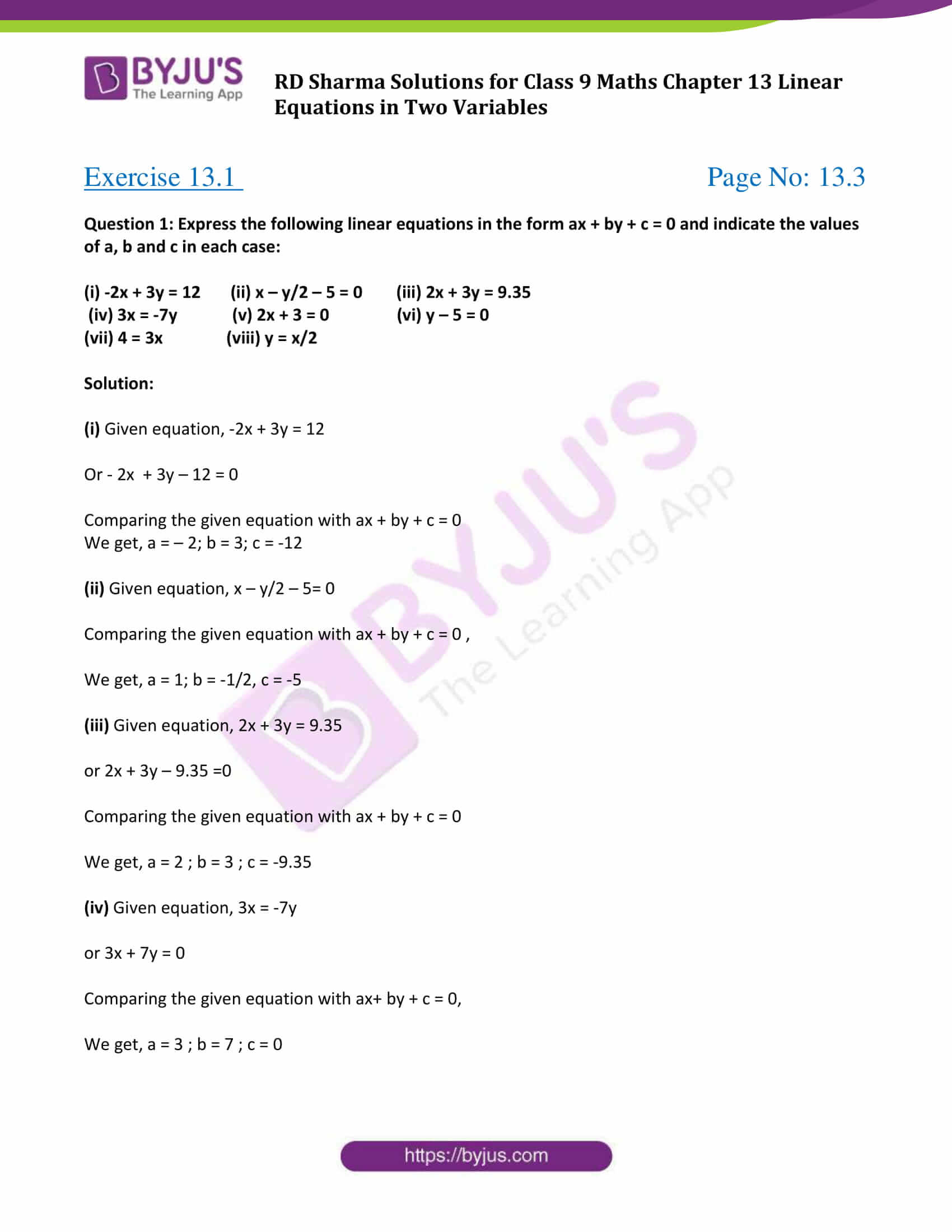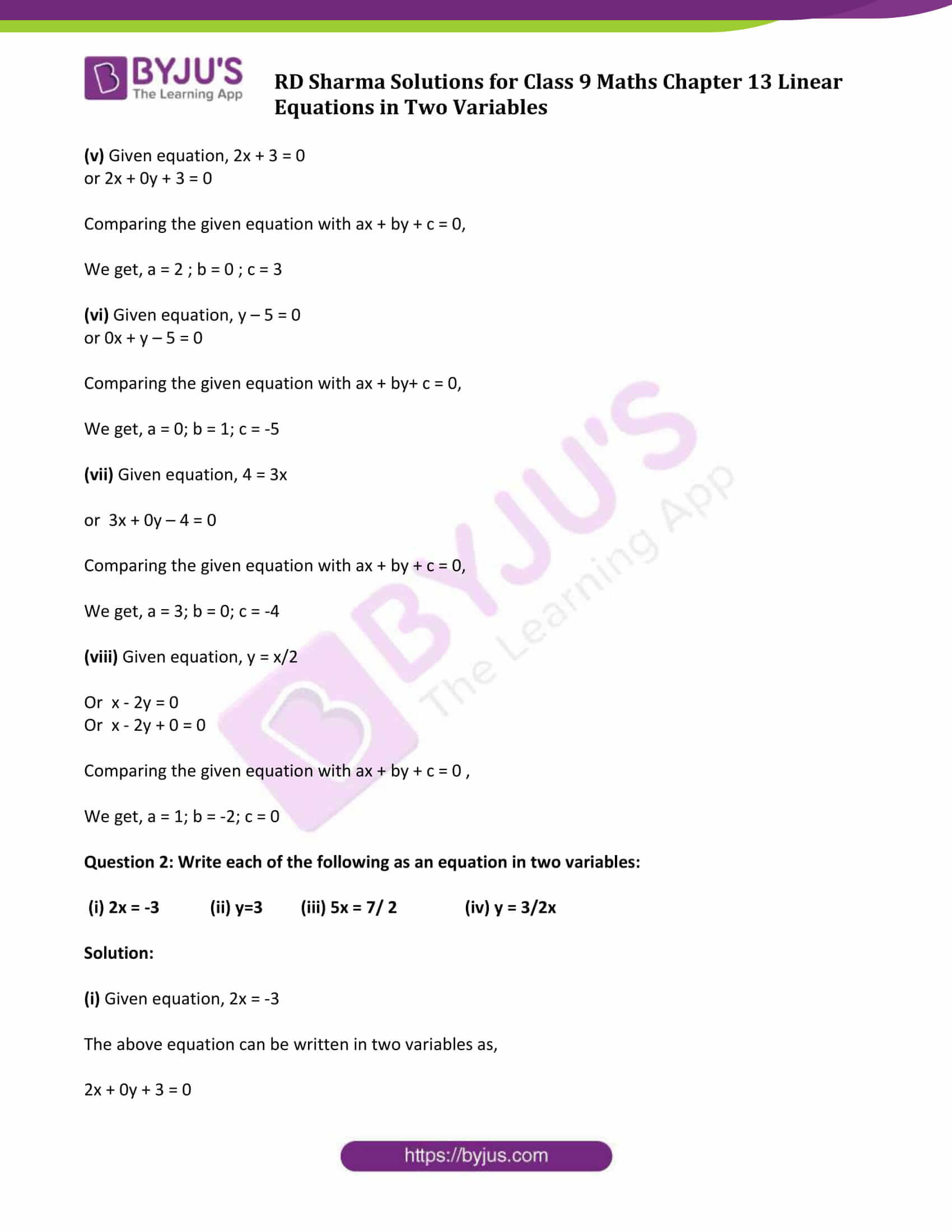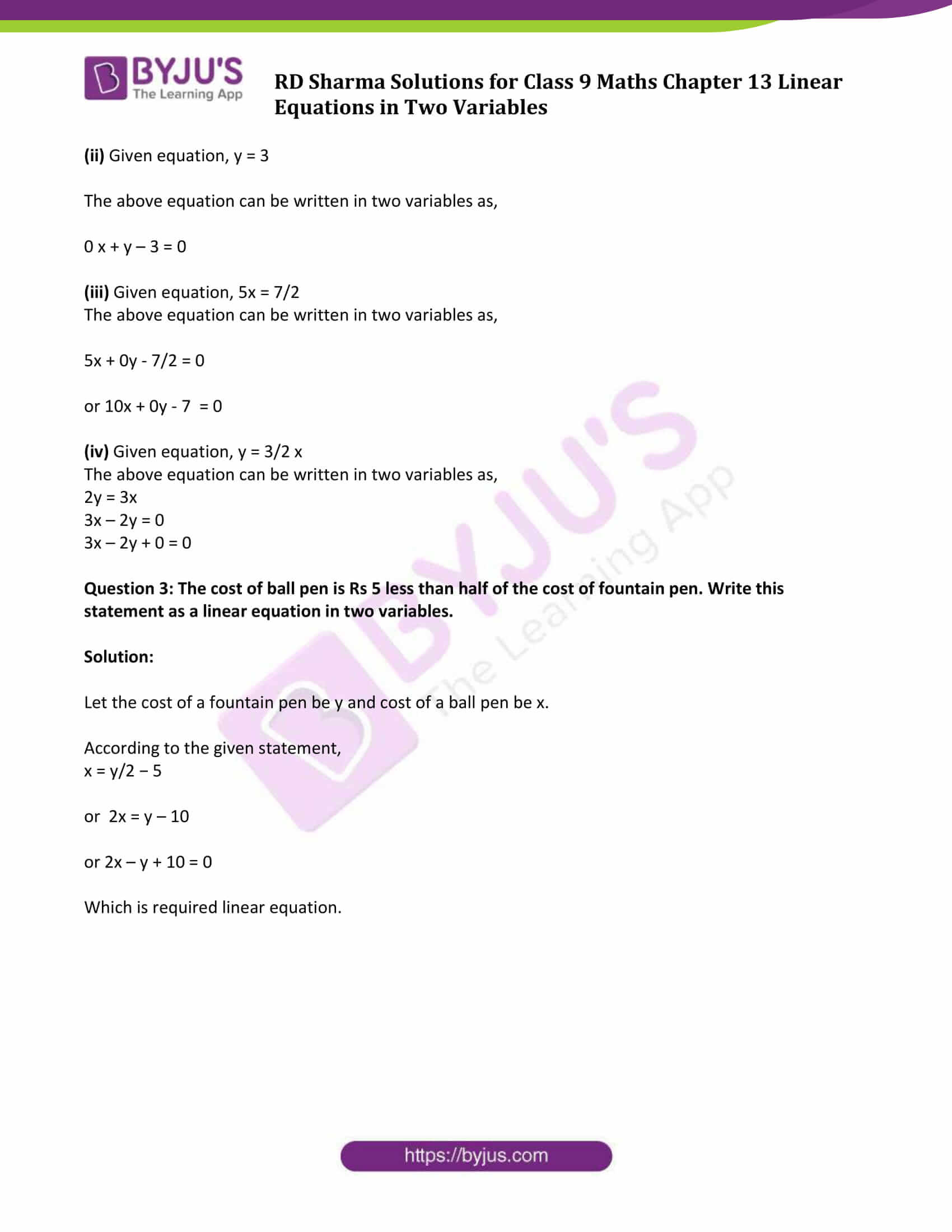# RD Sharma Solutions for Class 9 Maths Chapter 13 Linear Equations in Two Variables Exercise 13.1

This exercise mainly deal with the equations with degree one which are known as linear equations. ax + by + c = 0 is the standard form of a linear equation in two variables, (where a and b are real numbers and a, b are not equal to 0). In a linear equation, the same number can be added or subtracted from both sides of the equation and both sides can be multiplied or divided by the same non zero number. Students can download RD Sharma solution class 9 chapter 13 exercise 13.1 from the link given below.

## Download PDF of RD Sharma Solutions for Class 9 Maths Chapter 13 Linear Equations in Two Variables Exercise 13.1### Access Answers to Maths RD Sharma Solutions for Class 9 Chapter 13 Linear Equations in Two Variables Exercise 13.1 Page number 13.3

Question 1: Express the following linear equations in the form ax + by + c = 0 and indicate the values of a, b and c in each case:

(i) -2x + 3y = 12 (ii) x – y/2 – 5 = 0 (iii) 2x + 3y = 9.35

(iv) 3x = -7y (v) 2x + 3 = 0 (vi) y – 5 = 0

(vii) 4 = 3x (viii) y = x/2

Solution:

(i) Given equation, -2x + 3y = 12

Or – 2x + 3y – 12 = 0

Comparing the given equation with ax + by + c = 0

We get, a = – 2; b = 3; c = -12

(ii) Given equation, x – y/2 – 5= 0

Comparing the given equation with ax + by + c = 0 ,

We get, a = 1; b = -1/2, c = -5

(iii) Given equation, 2x + 3y = 9.35

or 2x + 3y – 9.35 =0

Comparing the given equation with ax + by + c = 0

We get, a = 2 ; b = 3 ; c = -9.35

(iv) Given equation, 3x = -7y

or 3x + 7y = 0

Comparing the given equation with ax+ by + c = 0,

We get, a = 3 ; b = 7 ; c = 0

(v) Given equation, 2x + 3 = 0

or 2x + 0y + 3 = 0

Comparing the given equation with ax + by + c = 0,

We get, a = 2 ; b = 0 ; c = 3

(vi) Given equation, y – 5 = 0

or 0x + y – 5 = 0

Comparing the given equation with ax + by+ c = 0,

We get, a = 0; b = 1; c = -5

(vii) Given equation, 4 = 3x

or 3x + 0y – 4 = 0

Comparing the given equation with ax + by + c = 0,

We get, a = 3; b = 0; c = -4

(viii) Given equation, y = x/2

Or x – 2y = 0

Or x – 2y + 0 = 0

Comparing the given equation with ax + by + c = 0 ,

We get, a = 1; b = -2; c = 0

Question 2: Write each of the following as an equation in two variables:

(i) 2x = -3 (ii) y=3 (iii) 5x = 7/ 2 (iv) y = 3/2x

Solution:

(i) Given equation, 2x = -3

The above equation can be written in two variables as,

2x + 0y + 3 = 0

(ii) Given equation, y = 3

The above equation can be written in two variables as,

0 x + y – 3 = 0

(iii) Given equation, 5x = 7/2

The above equation can be written in two variables as,

5x + 0y – 7/2 = 0

or 10x + 0y – 7 = 0

(iv) Given equation, y = 3/2 x

The above equation can be written in two variables as,

2y = 3x

3x – 2y = 0

3x – 2y + 0 = 0

Question 3: The cost of ball pen is Rs 5 less than half of the cost of fountain pen. Write this statement as a linear equation in two variables.

Solution:

Let the cost of a fountain pen be y and cost of a ball pen be x.

According to the given statement,

x = y/2 − 5

or 2x = y – 10

or 2x – y + 10 = 0

Which is required linear equation.

## RD Sharma Solutions for Class 9 Maths Chapter 13 Linear Equations in Two Variables Exercise 13.1

Class 9 Maths Chapter 13 Linear Equations in Two Variables Exercise 13.1 is based on brief introduction of linear equations in two variables. The RD Sharma solutions given here are for class 9 are includes the solutions for all the questions enlisted under exercise.